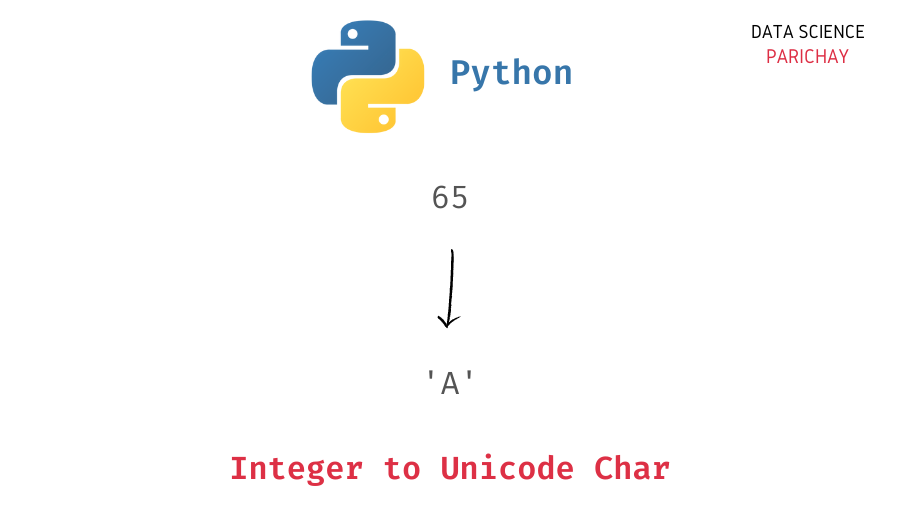# Convert Integer to Char in Python

In this tutorial, we will look at how to convert an integer to a char in Python with the help of some examples. We’ll be looking at use-cases of finding the char (string) representing a character whose Unicode point is the integer.

## How to convert integer to char in Python?

You can use the Python built-in `chr()` function to get the character represented by a particular integer in Unicode. The following is the syntax –

`chr(i)`

Pass the integer as an argument. The valid range for the integer argument is from 0 through 1,114,111 (0x10FFFF in base 16). If you pass an integer outside of this range, it will result in a `ValueError`.

## Examples

Let’s look at some examples of using the above syntax –

### Get the character represented by a number in Unicode

Let’s pass the integer 65 to the `chr()` function.

```# integer to char
ch = chr(65)
# display char and its type
print(ch)
print(type(ch))```

Output:

```A
<class 'str'>```

We get `'A'` from the function. The number 65 points to the character `'A'` in Unicode.

Let’s look at another example.

📚 Data Science Programs By Skill Level

Introductory

Intermediate ⭐⭐⭐

🔎 Find Data Science Programs 👨‍💻 111,889 already enrolled

Disclaimer: Data Science Parichay is reader supported. When you purchase a course through a link on this site, we may earn a small commission at no additional cost to you. Earned commissions help support this website and its team of writers.

```# integer to char
ch = chr(44)
# display char and its type
print(ch)
print(type(ch))```

Output:

```,
<class 'str'>```

We get the comma character, `','` for the integer 44.

You can use the Python built-in `ord()` function to get the corresponding integer for a character in Unicode.

```# char to integer
print(ord('A'))
print(ord(','))```

Output:

```65
44```

We get 65 for the character ‘A’ and 26 for the character ‘\$’.

### What if you pass a negative integer to the above function?

Let’s pass a negative integer to the `chr()` function as an argument.

```# integer to char
ch = chr(-65)
# display char and its type
print(ch)
print(type(ch))```

Output:

```---------------------------------------------------------------------------
ValueError                                Traceback (most recent call last)
Input In , in <module>
1 # integer to char
----> 2 ch = chr(-65)
3 # display char and its type
4 print(ch)

ValueError: chr() arg not in range(0x110000)```

We get a `ValueError`. This is because the passed integer is outside the valid range of 0 through 1,114,111.

You might also be interested in –

•